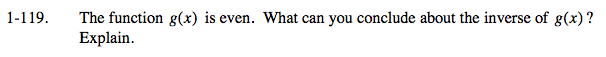### Home > CALC > Chapter 1 > Lesson 1.3.2 > Problem1-119

1-119.

The function g(x) is even. What can you conclude about the inverse of g(x) ? Explain. Homework Help ✎Think about a famous even functions, like y = x2. Visualize the graph of its inverse,

What is significant about the ± aspect of the graph?

Is the inverse of an even function also a function? That is, are there any x-values that have two corresponding y-values?